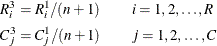#### Definitions and Notation

A two-way table represents the crosstabulation of row variable X and column variable Y. Let the table row values or levels be denoted by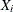,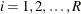, and the column values by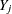,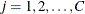. Let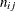denote the frequency of the table cell in the ith row and jth column and define the following notation: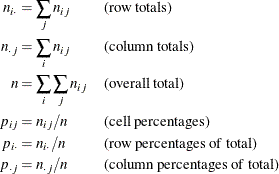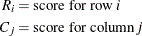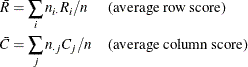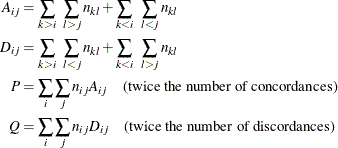##### Scores

PROC FREQ uses scores of the variable values to compute the Mantel-Haenszel chi-square, Pearson correlation, Cochran-Armitage test for trend, weighted kappa coefficient, and Cochran-Mantel-Haenszel statistics. The SCORES= option in the TABLES statement specifies the score type that PROC FREQ uses. The available score types are TABLE, RANK, RIDIT, and MODRIDIT scores. The default score type is TABLE. Using MODRIDIT, RANK, or RIDIT scores yields nonparametric analyses.

For numeric variables, table scores are the values of the row and column levels. If the row or column variable is formatted, then the table score is the internal numeric value corresponding to that level. If two or more numeric values are classified into the same formatted level, then the internal numeric value for that level is the smallest of these values. For character variables, table scores are defined as the row numbers and column numbers (that is, 1 for the first row, 2 for the second row, and so on).

Rank scores, which you request with the SCORES=RANK option, are defined as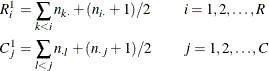where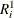is the rank score of row i, and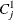is the rank score of column j. Note that rank scores yield midranks for tied values.

Ridit scores, which you request with the SCORES=RIDIT option, are defined as rank scores standardized by the sample size (Bross, 1958; Mack and Skillings, 1980). Ridit scores are derived from the rank scores as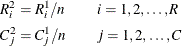Modified ridit scores (SCORES=MODRIDIT) represent the expected values of the order statistics of the uniform distribution on (0,1) (van Elteren, 1960; Lehmann and D’Abrera, 2006). Modified ridit scores are derived from rank scores as